Open in App
Not now

# Designing Finite Automata from Regular Expression (Set 6)

• Difficulty Level : Basic
• Last Updated : 20 Nov, 2019

In the below article, we shall see some Designing of Finite Automata form the given Regular Expression-

Regular Expression 1: Regular language,

`L1 = a(a+b)* `

The language of the given RE is,

`{aaa, aba, baa, bba} `

Its finite automata will be like below-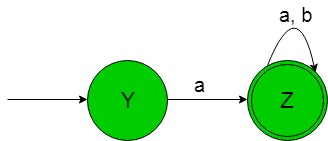In the above transition diagram, as we can see that initial state ‘Y’ on getting ‘a’ as the input it transits to a
final state ‘Z’ and so on for the remaining states. Thus this FA accepting all the strings of the given RE language.

Regular Expression 2: Regular language,

`L2 = (a+b)*a `

The language of the given RE is,

`{aaa, aba, baa, bba} `

Strings always end with ‘a’.
Its finite automata will be like below-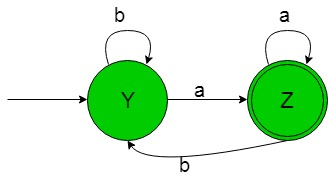In the above transition diagram, as we can see that initial state ‘Y’ on getting ‘a’ as the input it transits to a final state ‘Z’ and on getting ‘b’ as the input it remains in the state of itself and so on for the remaining states. Thus this FA accepting all the strings of the given RE language.

Regular Expression 3: Regular language,

`L3 = (a+b)*a(a+b)* `

The language of the given RE is,

`{aaa, aba, baa, bba} `

Strings containing ‘a’ as the alphabet.
Its finite automata will be like below-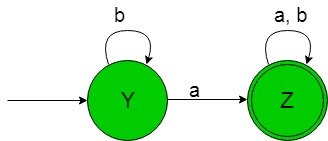In the above transition diagram, as we can see that initial state ‘Y’ on getting ‘b’ as the input it remains in the state of itself and on getting ‘a’ as the input it transits to a final state ‘Z’ and so on for the remaining states. Thus this FA accepting all the strings of the given RE language.

Regular Expression 4: Regular language,

`L4 = (a(a+b)*b)+(b(a+b)*a) `

The language of the given RE is,

`{aab, abb, baa, bba} `

Strings start and end with different symbols.
Its finite automata will be like below-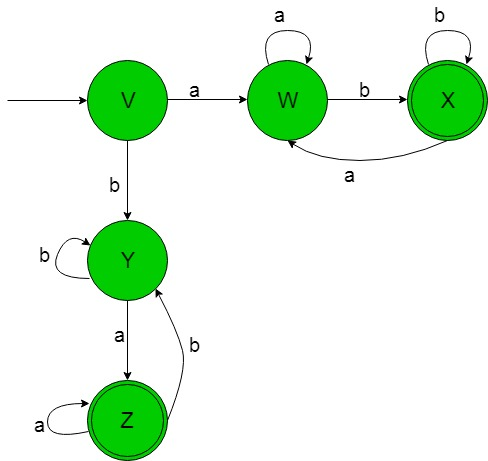In the above transition diagram, as we can see that initial state ‘V’ on getting ‘a’ as the input it transits to a state ‘W’ and so on for the remaining states. Thus this FA accepting all the strings of the given RE language.

Regular Expression 5: Regular language,

`L5 = (a(a+b)*a)+(b(a+b)*b)+a+b+ε `

The language of the given RE is,

`{&epsilon, a, b, aba, bab, bbab} `

Strings start and end with same symbols.
Its finite automata will be like below-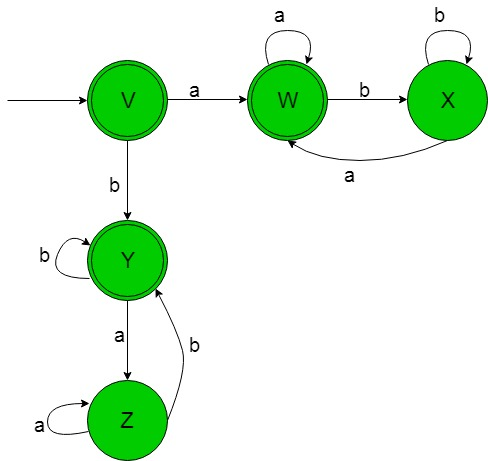In the above transition diagram, as we can see that initial and final state ‘V’ on getting ‘a’ as the input it transits to another final state ‘W’ and so on for the remaining states. Thus this FA accepting all the strings of the given RE language.

My Personal Notes arrow_drop_up